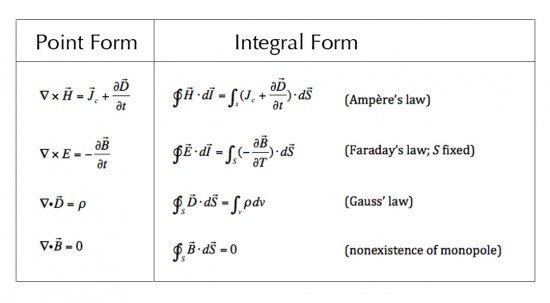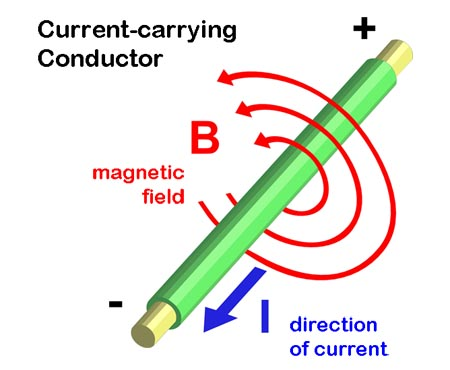## Appendix II: The Electro-magnetic Field EquationsIntroduction Maxwell’s Equations and the Lorentz Force Law together comprise the e/m field equations; i.e., those equations determining the interactions of charged particles in the vicinity of electric and magnetic fields and the resultant effect of those interactions on the values of the e/m field. For ease of explanation, the…

## Essential Guide to the EU – Chapter 2 Magnetic and Electric Fields in Space2.1 The Strength of Gravity and Electric Forces Gravity is a relatively very weak force.  The electric Coulomb force between a proton and an electron is of the order of 1039 (that’s 1 with 39 zeros after it) times stronger than the gravitational force between them. We can get a…